# 90.## Creating Custom Functions

Once you assign a value to a variable, you can use that value in any expression. For example, after you assign the number 25 to the variable myAge, the value of the conditional expression myAge > 20 would be True because 25 is greater than 20. You use the variable as if it were the value that it contains.

A function is like a variable, except that a function is smarter. A function is a variable that figures out its own value whenever you use it. For example, Microsoft Visual Basic has a function named Time. When you use the conditional expression Time > #8:00 PM#, the Time function checks the time on your computer's clock each time you use the expression.

Visual Basic has many built-in functions. Excel also has many built-in functions. Those functions are useful, but they aren't customizable. Even if you find a Visual Basic function that's 'this close' to what you need, you can't worm your way into the innards of Visual Basic to change the way it works. You can, however, create a function of your own. Because your function can take advantage of any of the Excel or Visual Basic built-in functions, and because you can customize your function however you want, you get the same benefit you would get if you could tweak the built-in functions directly.

Use a Custom Function from a Worksheet

Both Excel and Visual Basic have functions that return a random number between 0 and 1. The Excel function is named RAND(), and the Visual Basic function is named Rnd. You can use the Excel function in a worksheet cell, but you can use the Visual Basic function only in a macro.

You can't customize the Visual Basic Rnd function or the Excel RAND()function, but you can create a custom random-number function-let's call it Random-that you can use from Excel. Why would you want to create your own random-number function when you could use Excel's built-in one for free? Because you want your Random function to behave just a little differently than Excel's. Once you create your own function, you can make it do whatever you want.

1. Enter the formula =Random() into cell A3 on the TestFunction sheet.

Excel displays the #NAME? error value because the Random function doesn't exist yet.Run Macro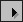2. Click the Run Macro button, type Random in the Macro Name box, and then click Create.

3. Double-click the word Sub at the beginning of the macro, and replace it with Function.

The End Sub statement changes to End Function. You've now created a function. Next you need to tell Excel what to use as the value of the function.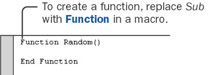4. Type the statement Random = Rnd as the body of the function. The revised function should look like this:

`Function Random()     Random = Rnd End Function`

The way you tell a function what value to return is by assigning a value to the name of the function, as if the function name were a variable. This function simply takes the value of the Visual Basic Rnd function and assigns it to the Random function.

Insert Function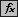5. Switch back to Excel, select cell A3, and then click the Insert Function button next to the formula bar. Excel displays the Function Arguments window, which explains that the Random function doesn't take any arguments. Click OK to enter the random number into cell A3.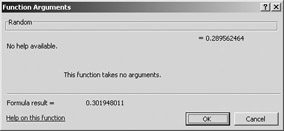That's all there is to creating a simple worksheet function. In the Visual Basic Editor, you replace the word Sub with Function, and then somewhere in the function, you assign a value to the function name. In Excel, you put the function name into a formula, followed by parentheses.

Add Arguments to a Custom Function

Suppose that you want random whole numbers equal to 100 plus or minus 25. Or that you want random whole numbers equal to 1000 plus or minus 100. The Excel RAND()function can't give you that kind of random number. Neither, for that matter, can yours, but because yours is a custom function, you can add capabilities to it by adding arguments.

To specify random-number ranges such as those just mentioned, you need three arguments: one to specify the midpoint, one to specify the plus or minus range, and one to specify whether or not to round the final number. You can add those arguments to your function.

1. In the Visual Basic Editor, type Midpoint, Range, Round between the parentheses after the name of the function. The statement that contains the function name and its arguments is called the function declaration statement. In this statement, you declare the name of the function and also the names of all the arguments. The revised function declaration statement should look like this:

`Function Random(Midpoint, Range, Round)`

These three words are arguments to the function. You can use them inside the function as variables that have been prefilled with values.

2. Change the statement that assigns a value to the function name to this:

`Random = Rnd * (Range * 2) + (Midpoint-Range)`

The Rnd function returns a random number between 0 and 1. If Range is equal to 25, that means you want numbers from 25 below the midpoint to 25 above the midpoint, for a total range of 50. Multiplying Rnd by Range * 2 would then give you a random number between 0 and 50. If the target midpoint is 100, you need to add 75 (that is, 100-25), to the random number. That's what this statement does.

3. Insert these three statements to round the number if requested:

`If Round Then     Random = CLng(Random) End If`

In Visual Basic, a Long is a whole number that can include large numbers. The Visual Basic function CLng converts a number to a Long, rounding it along the way. You round the random number only if the value of the Round argument is True. (Because the value of the Round argument already equals True or False, you don't need to compare it to anything to get a conditional expression.) The complete function should look like this:

`Function Random(Midpoint, Range, Round)     Random = Rnd * (Range * 2) + (Midpoint-Range)     If Round Then         Random = CLng(Random)     End If  End Function`
 Tip To see other functions that convert between data types, click CLng and press F1.
4. In Excel, enter 100 into cell B3, 25 into cell C3, and TRUE into cell D3. You'll use these values for the Midpoint, Range, and Round arguments of your function.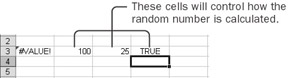Insert Function5. Select cell A3, and click the Insert Function button next to the formula bar.

The Function Arguments window appears, showing you the three new arguments of your function.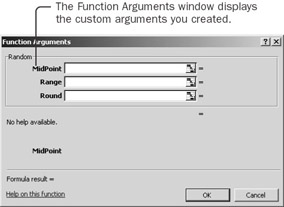6. Click in the Midpoint box, and then click in cell B3. Click in the Range box, and then click in cell C3. Click in the Round box, and then click in cell D3. Then click OK.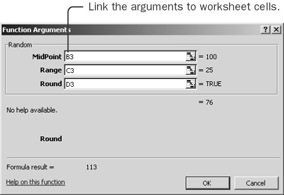After adjusting the formula, cell A3 contains a random number between 75 and 125. You use arguments to pass values to a function.

7. Change cell B3 to 1000 and cell C3 to 100. The value of cell A3 changes to a random number between 900 and 1100. Whenever you change the value of a cell that the function refers to, the function calculates a new answer. Adding arguments is a way to make functions more flexible.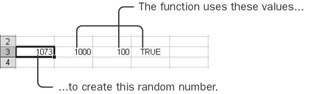Make a Function Volatile

Most functions recalculate only when the value of a cell that feeds into the function changes. Other functions (such as Excel's RAND()function), called volatile functions, recalculate whenever any cell on the worksheet changes or whenever you press F9. You can make your function volatile; it will then calculate a new random number whenever you press F9.

1. In Excel, press F9 repeatedly to see that the random number in cell A3 doesn't change.

2. In the Visual Basic Editor, insert this statement after the statement containing the name of the function:

`Application.Volatile True`
3. Switch back to Excel, and press F9.

The random number in cell A3 changes. Press F9 several times to verify that the function generates random numbers in the appropriate range.

Most of the time, you don't want custom functions to be volatile. You want the function to recalculate only when a value that feeds into it changes. For those few cases in which you do want the formula to recalculate, just use the Application object's Volatile method with True as an argument.

Make Arguments Optional

The only problem with your new enhanced Random function is that it's now more complicated to use in those simple cases in which you don't need the new arguments. If you put =Random() into a cell, omitting the arguments, Excel displays the #VALUE! error value. To avoid this error, you can tell Visual Basic that you want the arguments to be optional. Then you specify default values to use if the argument isn't supplied.

1. In the Visual Basic Editor, type the word Optional in front of each of the three argument names. The revised statement should look like this:

`Function Random(Optional Midpoint, _     Optional Range, Optional Round)`

You don't have to make all the arguments optional, but once you make one argument optional, all the arguments that follow it must be optional as well. In other words, you place optional arguments at the end of the argument list.

2. Type = 0.5 after the word Midpoint, = 0.5 after the word Range, and = False after the word Round. Break the statement into two lines after the first comma. The resulting statement should look like this:

`Function Random(Optional Midpoint = 0.5, _     Optional Range = 0.5, Optional Round = False)`

You can specify a default value for any optional argument. You assign the default value to the argument name in the same way you would assign a value to a variable-by using a simple equal sign.

3. In Excel, enter =Random() into cell A4. A random number between 0 and 1 appears.

4. Delete the formulas in cells A3 and A4 so that you can step through other macros later in the chapter without stepping through the custom function.

Optional arguments allow you to add powerful features to a function while keeping it easy to use in cases in which you don't need the extra features. To make an argument optional, add Optional before the argument name. To add a default value for an optional argument, assign the value to the argument name the same way you would if it were a variable.

Use a Custom Function from a Macro

You can use a custom function from a macro just as easily as you can use it from a worksheet cell.

1. In the Visual Basic Editor, type Sub TestRandom at the bottom of the module, and then press the Enter key to start creating a macro.

2. Type MsgBox and a space.

Visual Basic shows the Quick Info box with the arguments for MsgBox.

3. Press Ctrl+Spacebar to show the list of global methods and properties, and then press R to scroll down to the words that begin with an R.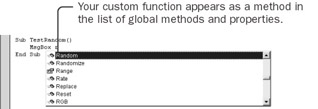Your Random function is automatically included in the list. Your function has the icon for a method next to it. Excel methods are simply functions built into Excel. You create new global methods simply by writing new functions.

4. Press the Tab key to insert the function name into the statement, and then type an opening parenthesis to begin the argument list. Visual Basic displays the Quick Info box with the arguments for your custom function. The Quick Info box even shows the default values for the optional arguments.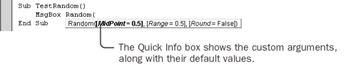5. Type 200, 5, True as the list of arguments, and then type a closing parenthesis.

6. Press F5 to run the macro. Click OK when your random number appears.A function is a procedure like a Sub procedure, except that it returns a value that you can use either in a cell in Excel or from a macro.

 Important A function used in a worksheet cell can include only those actions that can be executed while Excel is recalculating a worksheet. (Remember that some cells might even recalculate more than once.) Actions such as opening files or displaying message boxes can be included in functions that are called from macros, but if you include them in a function that's called from a worksheet, the function simply returns the #VALUE! error value.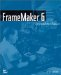FrameMaker 6: Beyond the Basics
ISBN: 735613591
EAN: 2147483647
Year: 2005
Pages: 122
Authors: Lisa Jahred

Similar book on Amazon